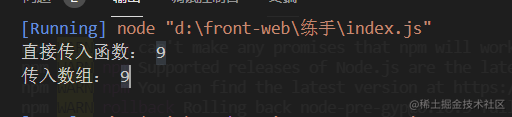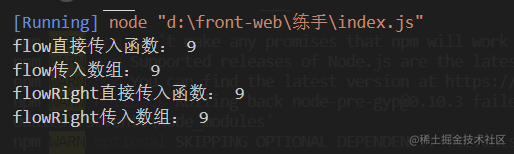前言

啥是洋葱代码像洋葱的切面一样一层一层的嵌套在一起的代码 '(当然还有面条式代码，这种代码才是最垃圾的，千万避免)'

让我们来一个实际一点的案例

_.toUpper(_.first(_.reverse(array)))

管道

管道拆分

Q： 管道的长度、广度过大的时候，如果这个管道出现了一些纰漏，那我们排查起来的工作量就很大，很困难，该如何处理呢？

A： 拆分管道！

fn = compose(f1,f2,f3)
b = fn(a)

函数组合(compose)

什么是函数组合

• 函数就像是数据管道，而函数组合就是把这些进行连接起来，让数据穿过多个管道形成最终结果
• 函数组合默认是从右到左执行

lodash中的组合函数

flow

1. [funcs] (...(Function|Function[])) : 要调用的函数。

(Function) （ 返回新的函数。） 例子

// 引入lodash
const _ = require('lodash')

function square(n) {
return n * n;
}
return augend + augend;
}
// 当然也可以这样使用

flowRight

1. [funcs] (...(Function|Function[])) : 要调用的函数。

(Function) （ 返回新的函数。）

// 引入lodash
const _ = require('lodash')

function square(n) {
return n * n;
}
return augend + augend1;
}
// 当然也可以这样使用

手写函数组合demo

• 函数可以接收参数，如果是数组的话，有且只能有一个，如果不为数组的话参数的数量不限
• 函数需要返回一个函数，且我们返回的函数要对数据进行处理

ok带着这些需求我们先整理一份简单的demo出来吧

1.获取参数

// 老规矩es6的剩余参数
function flow(...funs) {
console.log(funs)
}

ok,我们第一步已经完成了，不过有几个问题，我们如果传入flow(方法一，方法2) 打印出来的结果会是[方法一，方法2],但是我们传入flow([方法一，方法2])打印出来的结果会是[[方法一，方法2]],这样对我们的之后进行不是很方便，我们稍微做一下数组的扁平化吧！

2.数组扁平化

function flatten(arr) {
return arr.reduce((result, item)=> {
return result.concat(Array.isArray(item) ? flatten(item) : item);
}, []);
}

function flatten(arr) {
return arr.reduce((result, item)=> {
return result.concat(Array.isArray(item) ? [...item] : item);
}, []);
}

3.第三步 完结版本

// 老规矩es6的剩余参数
function flow(...funs) {
// 这里我们要进行一下判断中是否都是方法体
let funList = flatten(funs)
const length = funList.length
for(const fun of funList){
if (typeof fun !== 'function') {
throw new TypeError('Expected a function')
}
}
return function(...args) {
let index = 0
let result = length ? funList[index].apply(this, args) : args
while (++index < length) {
result = funList[index].call(this, result)
}
return result
}
}

测试小小的优化

function create(isRight){
return function (...funs) {
let funList = flatten(funs)
if(isRight){
funList.reverse();
}
// 这里我们要进行一下判断中是否都是方法体

const length = funList.length
for(const fun of funList){
if (typeof fun !== 'function') {
throw new TypeError('Expected a function')
}
}
return function(...args) {
let index = 0
let result = length ? funList[index].apply(this, args) : args
while (++index < length) {
result = funList[index].call(this, result)
}
return result
}
}
}

测试一下

const flow = create()
const flowRight = create(true)完整代码

function square(n) {
return n * n;
}
return augend + augend1;
}
function flatten(arr) {
return arr.reduce((result, item)=> {
return result.concat(Array.isArray(item) ? [...item] : item);
}, []);
}
function create(isRight){
return function (...funs) {
let funList = flatten(funs)
if(isRight){
funList.reverse();
}
// 这里我们要进行一下判断中是否都是方法体
const length = funList.length
for(const fun of funList){
if (typeof fun !== 'function') {
throw new TypeError('Expected a function')
}
}
return function(...args) {
let index = 0
let result = length ? funList[index].apply(this, args) : args
while (++index < length) {
result = funList[index].call(this, result)
}
return result
}
}
}
const flow = create()
const flowRight = create(true)

小结